Paul's Online Notes
Home / Calculus I / Applications of Derivatives / More Optimization Problems
Show Mobile Notice Show All Notes Hide All Notes
Mobile Notice
You appear to be on a device with a "narrow" screen width (i.e. you are probably on a mobile phone). Due to the nature of the mathematics on this site it is best views in landscape mode. If your device is not in landscape mode many of the equations will run off the side of your device (should be able to scroll to see them) and some of the menu items will be cut off due to the narrow screen width.

### Section 4.9 : More Optimization

Because these notes are also being presented on the web we’ve broken the optimization examples up into several sections to keep the load times to a minimum. Do not forget the various methods for verifying that we have the optimal value that we looked at in the previous section. In this section we’ll just use them without acknowledging so make sure you understand them and can use them. So let’s get going on some more examples.

Example 1 A window is being built and the bottom is a rectangle and the top is a semicircle. If there is 12 meters of framing materials what must the dimensions of the window be to let in the most light?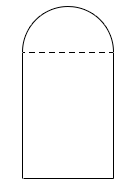Show Solution

Okay, let’s ask this question again in slightly easier to understand terms. We want a window in the shape described above to have a maximum area (and hence let in the most light) and have a perimeter of 12 m (because we have 12 m of framing material). Little bit easier to understand in those terms.

Let the radius of the semicircle on the top be $$r$$ and the height of the rectangle be $$h$$. Now, because the semicircle is on top of the window we can think of the width of the rectangular portion at 2$$r$$ as shown below.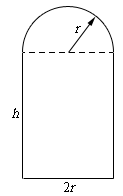The perimeter (our constraint) is the lengths of the three sides on the rectangular portion plus half the circumference of a circle of radius $$r$$. The area (what we want to maximize) is the area of the rectangle plus half the area of a circle of radius $$r$$. Here are the equations we’ll be working with in this example.

\begin{align*}{\mbox{Maximize : }} & A = 2hr + {\frac{1}{2}}\pi {r^2}\\ {\mbox{Constraint :}} & 12 = 2h + 2r + \pi r\end{align*}

In this case we’ll solve the constraint for $$h$$ and plug that into the area equation.

$h = 6 - r - {\frac{1}{2}}\pi r\hspace{0.5in} \Rightarrow \hspace{0.25in}A\left( r \right) = 2r\left( {6 - r - {\frac{1}{2}}\pi r} \right) + {\frac{1}{2}}\pi {r^2} = 12r - 2{r^2} - {\frac{1}{2}}\pi {r^2}$

The first and second derivatives are,

$A'\left( r \right) = 12 - r\left( {4 + \pi } \right)\hspace{0.75in}A''\left( r \right) = - 4 - \pi$

We can see that the only critical point is,

$r = \frac{{12}}{{4 + \pi }} = 1.6803$

We can also see that the second derivative is always negative (in fact it’s a constant) and so we can see that the maximum area must occur at this point. So, for the maximum area the semicircle on top must have a radius of 1.6803 and the rectangle must have the dimensions 3.3606 x 1.6803 $$(2r$$ x $$h)$$ .

Example 2 Determine the area of the largest rectangle that can be inscribed in a circle of radius 4.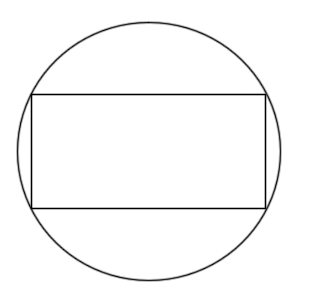Show Solution

Huh? This problem type of problem never seems to make sense originally. What we want to do is maximize the area of the largest rectangle that we can fit inside a circle and have all of its corners touching the circle.

To do this problem it’s easiest to assume that the circle (and hence the rectangle) is centered at the origin of a standard $$xy$$ axis system. Doing this we know that the equation of the circle will be

${x^2} + {y^2} = 16$

and that the right upper corner of the rectangle will have the coordinates $$\left( {x,y} \right)$$. This means that the width of the rectangle will be 2$$x$$ and the height of the rectangle will be 2$$y$$ as shown below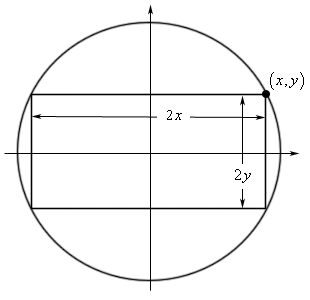The area of the rectangle will then be,

$A = \left( {2x} \right)\left( {2y} \right) = 4xy$

So, we’ve got the function we want to maximize (the area), but what is the constraint? Well since the coordinates of the upper right corner must be on the circle we know that $$x$$ and $$y$$ must satisfy the equation of the circle. In other words, the equation of the circle is the constraint.

The first thing to do then is to solve the constraint for one of the variables.

$y = \pm \sqrt {16 - {x^2}}$

Since the point that we’re looking at is in the first quadrant we know that $$y$$ must be positive and so we can take the “+” part of this. Plugging this into the area and computing the first derivative gives,

\begin{align*}A\left( x \right) & = 4x\sqrt {16 - {x^2}} \\ A'\left( x \right) & = 4\sqrt {16 - {x^2}} - \frac{{4{x^2}}}{{\sqrt {16 - {x^2}} }} = \frac{{64 - 8{x^2}}}{{\sqrt {16 - {x^2}} }}\end{align*}

Before getting the critical points let’s notice that we can limit $$x$$ to the range $$0 \le x \le 4$$ since we are assuming that $$x$$ is in the first quadrant and must stay inside the circle. Now the four critical points we get (two from the numerator and two from the denominator) are,

$\begin{array}{lll}16 - {x^2} = 0 & \hspace{0.5in} \Rightarrow \hspace{0.5in} & x = \pm 4\\ 64 - 8{x^2} = 0 & \hspace{0.5in} \Rightarrow \hspace{0.5in} & x = \pm 2\sqrt 2 \end{array}$

We only want critical points that are in the range of possible optimal values so that means that we have two critical points to deal with : $$x = 2\sqrt 2$$ and $$x = 4$$. Notice however that the second critical point is also one of the endpoints of our interval.

Now, area function is continuous and we have an interval of possible solution with finite endpoints so,

$A\left( 0 \right) = 0\hspace{0.5in}A\left( {2\sqrt 2 } \right) = 32\hspace{0.5in}A\left( 4 \right) = 0$

So, we can see that we’ll get the maximum area if $$x = 2\sqrt 2$$ and the corresponding value of $$y$$ is,

$y = \sqrt {16 - {{\left( {2\sqrt 2 } \right)}^2}} = \sqrt 8 = 2\sqrt 2$

It looks like the maximum area will be found if the inscribed rectangle is in fact a square.

We need to again make a point that was made several times in the previous section. We excluded several critical points in the work above. Do not always expect to do that. There will often be physical reasons to exclude zero and/or negative critical points, however, there will be problems where these are perfectly acceptable values. You should always write down every possible critical point and then exclude any that can’t be possible solutions. This keeps you in the habit of finding all the critical points and then deciding which ones you actually need and that in turn will make it less likely that you’ll miss one when it is actually needed.

Example 3 Determine the point(s) on $$y = {x^2} + 1$$ that are closest to $$\left( {0,2} \right)$$.
Show Solution

Here’s a quick sketch of the situation.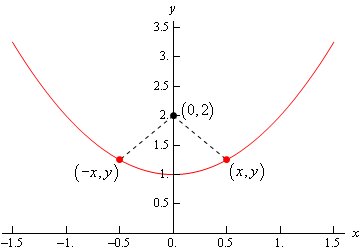So, we’re looking for the shortest length of the dashed line. Notice as well that if the shortest distance isn’t at$$x = 0$$ there will be two points on the graph, as we’ve shown above, that will give the shortest distance. This is because the parabola is symmetric to the $$y$$-axis and the point in question is on the $$y$$-axis. This won’t always be the case of course so don’t always expect two points in these kinds of problems.

In this case we need to minimize the distance between the point $$\left( {0,2} \right)$$ and any point that is one the graph $$\left( {x,y} \right)$$. Or,

$d = \sqrt {{{\left( {x - 0} \right)}^2} + {{\left( {y - 2} \right)}^2}} = \sqrt {{x^2} + {{\left( {y - 2} \right)}^2}}$

If you think about the situation here it makes sense that the point that minimizes the distance will also minimize the square of the distance and so since it will be easier to work with we will use the square of the distance and minimize that. If you aren’t convinced of this we’ll take a closer look at this after this problem. So, the function that we’re going to minimize is,

$D = {d^2} = {x^2} + {\left( {y - 2} \right)^2}$

The constraint in this case is the function itself since the point must lie on the graph of the function.

At this point there are two methods for proceeding. One of which will require significantly more work than the other. Let’s take a look at both of them.

Solution 1

In this case we will use the constraint in probably the most obvious way. We already have the constraint solved for $$y$$ so let’s plug that into the square of the distance and get the derivatives.

\begin{align*}D\left( x \right) & = {x^2} + {\left( {{x^2} + 1 - 2} \right)^2} = {x^4} - {x^2} + 1\\ D'\left( x \right) & = 4{x^3} - 2x = 2x\left( {2{x^2} - 1} \right)\\ D''\left( x \right) & = 12{x^2} - 2\end{align*}

So, it looks like there are three critical points for the square of the distance and notice that this time, unlike pretty much every previous example we’ve worked, we can’t exclude zero or negative numbers. They are perfectly valid possible optimal values this time.

$x = 0,\hspace{0.25in}x = \pm \frac{1}{{\sqrt 2 }}$

Before going any farther, let’s check these in the second derivative to see if they are all relative minimums.

$D''\left( 0 \right) = - 2 < 0\hspace{0.25in}D''\left( {\frac{1}{{\sqrt 2 }}} \right) = 4\hspace{0.5in}D''\left( { - \frac{1}{{\sqrt 2 }}} \right) = 4$

So, $$x = 0$$ is a relative maximum and so can’t possibly be the minimum distance. That means that we’ve got two critical points. The question is how we verify that these give the minimum distance and yes we did mean to say that both will give the minimum distance. Recall from our sketch above that if $$x$$ gives the minimum distance then so will –$$x$$ and so if gives the minimum distance then the other should as well.

None of the methods we discussed in the previous section will really work here. We don’t have an interval of possible solutions with finite endpoints and both the first and second derivative change sign. In this case however, we can still verify that they are the points that give the minimum distance.

First, let’s see what we have if we are working on the interval $$\left[ { - \frac{1}{\sqrt{2}},\frac{1}{\sqrt{2}}} \right]$$. On this interval we can try to use the first method of finding absolute extrema discussed in the previous section. That says to evaluate the function at the endpoints and the critical points and in this case, even though we’ve excluded it we’ll need to include $$x = 0$$ since it is a critical point in the region. Doing this gives,

$D\left( { - \frac{1}{\sqrt{2}}} \right) = {\frac{3}{4}}\hspace{0.5in}D\left( 0 \right) = 1\hspace{0.5in}D\left( \frac{1}{\sqrt{2}} \right) = {\frac{3}{4}}$

So, we can see that the absolute minimum in the interval must occur at $$x = \pm \frac{1}{\sqrt{2}}$$.

Next, we can see that if $$x < - \frac{1}{\sqrt{2}}$$ then $$D'\left( x \right) < 0$$. Or in other words, if $$x < - \frac{1}{\sqrt{2}}$$ the function is decreasing until it hits $$x = - \frac{1}{\sqrt{2}}$$ and so must always be larger than the function at $$x = - \frac{1}{\sqrt{2}}$$.

Similarly, $$x > \frac{1}{\sqrt{2}}$$ then $$D'\left( x \right) > 0$$ and so the function is always increasing to the right of $$x = \frac{1}{\sqrt{2}}$$ and so must be larger than the function at $$x = \frac{1}{\sqrt{2}}$$.

So, putting all of this together tells us that we do in fact have an absolute minimum at $$x = \pm \frac{1}{\sqrt{2}}$$.

All that we need to do is to find the value of $$y$$ for these points.

$\begin{array}{ll}x = \displaystyle \frac{1}{{\sqrt 2 }} & :\hspace{0.25in}y = \displaystyle \frac{3}{2}\\ x = - \displaystyle \frac{1}{{\sqrt 2 }} & :\hspace{0.25in}y = \displaystyle \frac{3}{2}\end{array}$

So, the points on the graph that are closest to $$\left( {0,2} \right)$$ are,

$\left( {\frac{1}{{\sqrt 2 }},\frac{3}{2}} \right)\hspace{0.25in}\left( { - \frac{1}{{\sqrt 2 }},\frac{3}{2}} \right)$

This solution method shows how tricky it can be to know that we have absolute extrema when there are multiple critical points and none of the methods discussed in the last section will work. Luckily for us, there is another, easier, method we could have done instead.

Solution 2

The first solution that we worked was actually the long solution. There is a much shorter, and easier, solution to this problem. Instead of plugging $$y$$ into the square of the distance let’s plug in $$x$$. From the constraint we get,

${x^2} = y - 1$

and notice that the only place $$x$$ show up in the square of the distance it shows up as $${x^2}$$ and let’s just plug this into the square of the distance. Doing this gives,

\begin{align*}D\left( y \right) & = y - 1 + {\left( {y - 2} \right)^2} = {y^2} - 3y + 3\\ D'\left( y \right) & = 2y - 3\\ D''\left( y \right) & = 2\end{align*}

There is now a single critical point, $$y = {\frac{3}{2}}$$, and since the second derivative is always positive we know that this point must give the absolute minimum. So, all that we need to do at this point is find the value(s) of $$x$$ that go with this value of $$y$$.

${x^2} = \frac{3}{2} - 1 = \frac{1}{2}\hspace{0.5in} \Rightarrow \hspace{0.5in}x = \pm \frac{1}{{\sqrt 2 }}$

The points are then,

$\left( {\frac{1}{{\sqrt 2 }},\frac{3}{2}} \right)\hspace{0.25in}\left( { - \frac{1}{{\sqrt 2 }},\frac{3}{2}} \right)$

So, for significantly less work we got exactly the same answer.

This previous example had a couple of nice points. First, as pointed out in the problem, we couldn’t exclude zero or negative critical points this time as we’ve done in all the previous examples. Again, be careful to not get into the habit of always excluding them as we do many of the examples we’ll work.

Next, some of these problems will have multiple solution methods and sometimes one will be significantly easier than the other. The method you use is up to you and often the difficulty of any particular method is dependent upon the person doing the problem. One person may find one way easier and other person may find a different method easier.

Finally, as we saw in the first solution method sometimes we’ll need to use a combination of the optimal value verification methods we discussed in the previous section.

Now, before we move onto the next example let’s take a look at the claim above that we could find the location of the point that minimizes the distance by finding the point that minimizes the square of the distance. We’ll generalize things a little bit,

#### Fact

Suppose that we have a positive function, $$f\left( x \right) > 0$$, that exists everywhere then $$f\left( x \right)$$ and $$g\left( x \right) = \sqrt {f\left( x \right)}$$ will have the same critical points and the relative extrema will occur at the same points.

This is simple enough to prove so let’s do that here. First let’s take the derivative of $$g\left( x \right)$$ and see what we can determine about the critical points of $$g\left( x \right)$$.

$g'\left( x \right) = {\frac{1}{2}}{\left[ {f\left( x \right)} \right]^{ - \,\,\frac{1}{2}}}\,\,f'\left( x \right) = \frac{{f'\left( x \right)}}{{2\sqrt {f\left( x \right)} }}$

Let’s plug $$x = c$$ into this to get,

$g'\left( c \right) = \frac{{f'\left( c \right)}}{{2\sqrt {f\left( c \right)} }}$

By assumption we know that $$f\left( c \right)$$ exists and $$f\left( c \right) > 0$$ and therefore the denominator of this will always exist and will never be zero. We’ll need this in several places so we can’t forget this.

If $$f'\left( c \right) = 0$$ then because we know that the denominator will not be zero here we must also have $$g'\left( c \right) = 0$$. Likewise, if $$g'\left( c \right) = 0$$ then we must have $$f'\left( c \right) = 0$$. So, $$f\left( x \right)$$ and $$g\left( x \right)$$ will have the same critical points in which the derivatives will be zero.

Next, if $$f'\left( c \right)$$ doesn’t exist then $$g'\left( c \right)$$ will also not exist and likewise if $$g'\left( c \right)$$ doesn’t exist then because we know that the denominator will not be zero then this means that $$f'\left( c \right)$$ will also not exist. Therefore, $$f\left( x \right)$$ and $$g\left( x \right)$$ will have the same critical points in which the derivatives does not exist.

So, the upshot of all this is that $$f\left( x \right)$$ and $$g\left( x \right)$$ will have the same critical points.

Next, let’s notice that because we know that $$2\sqrt {f\left( x \right)} > 0$$ then $$f'\left( x \right)$$ and $$g'\left( x \right)$$ will have the same sign and so if we apply the first derivative test (and recalling that they have the same critical points) to each of these functions we can see that the results will be the same and so the relative extrema will occur at the same points.

Note that we could also use the second derivative test to verify that the critical points will have the same classification if we wanted to. The second derivative is (and you should see if you can use the quotient rule to verify this),

$g''\left( x \right) = \frac{{2\sqrt {f\left( x \right)} \,f''\left( x \right) - {{\left[ {f'\left( x \right)} \right]}^2}{{\left[ {f\left( x \right)} \right]}^{ - \,\,\frac{1}{2}}}}}{{4f\left( x \right)}}$

Then if $$x = c$$ is a critical point such that $$f'\left( c \right) = 0$$ (and so we can use the second derivative test) we get,

\begin{align*}g''\left( c \right) & = \frac{{2\sqrt {f\left( c \right)} \,f''\left( c \right) - {{\left[ {f'\left( c \right)} \right]}^2}{{\left[ {f\left( c \right)} \right]}^{ - \,\,\frac{1}{2}}}}}{{4f\left( c \right)}}\\ & = \frac{{2\sqrt {f\left( c \right)} \,f''\left( c \right) - {{\left[ 0 \right]}^2}{{\left[ {f\left( c \right)} \right]}^{ - \,\,\frac{1}{2}}}}}{{4f\left( c \right)}} = \frac{{\sqrt {f\left( c \right)} \,f''\left( c \right)}}{{2f\left( c \right)}}\end{align*}

Now, because we know that $$2\sqrt {f\left( c \right)} > 0$$ and by assumption $$f\left( c \right) > 0$$ we can see that $$f''\left( c \right)$$ and $$g''\left( c \right)$$ will have the same sign and so will have the same conclusion from the second derivative test.

So, now that we have that out of the way let’s work some more examples.

Example 4 A 2 feet piece of wire is cut into two pieces and one piece is bent into a square and the other is bent into an equilateral triangle. Where, if anywhere, should the wire be cut so that the total area enclosed by both is minimum and maximum?
Show Solution

Before starting the solution recall that an equilateral triangle is a triangle with three equal sides and each of the interior angles are $${\frac{\pi}{3}}$$ (or $$60^\circ$$).

Also, note the “if anywhere” portion of the problem statement. What this is saying is that it is possible to take the full piece of wire and put all of it into either a square or a triangle. Do not forget about this as it will be important later on in the problem.

Now, this is another problem where the constraint isn’t really going to be given by an equation, it is simply that there is 2 ft of wire to work with and this will be taken into account in our work.

So, let’s cut the wire into two pieces. The first piece will have length $$x$$ which we’ll bend into a square and each side will have length $${\frac{x}{4}}$$. The second piece will then have length $$2 - x$$ (we just used the constraint here…) and we’ll bend this into an equilateral triangle and each side will have length $${\frac{1}{3}}\left( {2 - x} \right)$$. Here is a sketch of all this.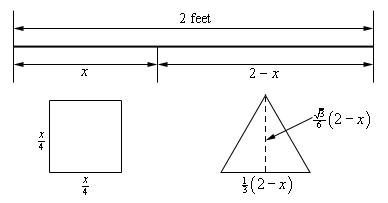As noted in the sketch above we also will need the height of the triangle. This is easy to get if you realize that the dashed line divides the equilateral triangle into two other triangles. Let’s look at the right one. The hypotenuse is $${\frac{1}{3}}\left( {2 - x} \right)$$ while the lower right angle is $${\frac{\pi}{3}}$$. Finally, the height is then the opposite side to the lower right angle so using basic right triangle trig we arrive at the height of the triangle as follows.

$\sin \left( {{\frac{\pi}{3}}} \right) = \frac{\rm{opp}}{\rm{hyp}} \hspace{0.25in} \Rightarrow \hspace{0.25in} \rm{opp} = {\frac{1}{3}}\left( {2 - x} \right)\sin \left( {{\frac{\pi}{3}}} \right) = {\frac{1}{3}}\left( {2 - x} \right)\left( \frac{\sqrt{3}}{2} \right) = \frac{\sqrt{3}}{6} \left( {2 - x} \right)$

So, the total area of both objects is then,

$A\left( x \right) = {\left( {{\frac{x}{4}}} \right)^2} + {\frac{1}{2}}\left( {{\frac{1}{3}}\left( {2 - x} \right)} \right)\left( \frac{\sqrt{3}}{6} {\left( {2 - x} \right)} \right) = \frac{x^{2}}{16} + \frac{\sqrt{3}}{36} {\left( {2 - x} \right)^2}$

Here’s the first derivative of the area.

$A'\left( x \right) = {\frac{x}{8}} + \frac{\sqrt{3}}{36} \left( 2 \right)\left( {2 - x} \right)\left( { - 1} \right) = {\frac{x}{8}} - \frac{\sqrt{3}}{9} + \frac{\sqrt{3}}{18} x$

Setting this equal to zero and solving gives the single critical point of,

$x = \frac{8\sqrt{3}}{9 + 4\sqrt{3}} = 0.8699$

Now, let’s notice that the problem statement asked for both the minimum and maximum enclosed area and we got a single critical point. This clearly can’t be the answer to both, but this is not the problem that it might seem to be.

Let’s notice that $$x$$ must be in the range $$0 \le x \le 2$$ and since the area function is continuous we use the basic process for finding absolute extrema of a function.

$A\left( 0 \right) = 0.1925\hspace{0.5in}A\left( {0.8699} \right) = 0.1087\hspace{0.5in}A\left( 2 \right) = 0.25$

So, it looks like the minimum area will arise if we take $$x = 0.8699$$ while the maximum area will arise if we take the whole piece of wire and bend it into a square.

As the previous problem illustrated we can’t get too locked into the answers always occurring at the critical points as they have to this point. That will often happen, but one of the extrema in the previous problem was at an endpoint and that will happen on occasion.

Example 5 A piece of pipe is being carried down a hallway that is 10 feet wide. At the end of the hallway the there is a right-angled turn and the hallway narrows down to 8 feet wide. What is the longest pipe that can be carried (always keeping it horizontal) around the turn in the hallway?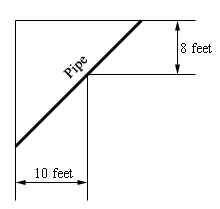Show Solution

Let’s start off with a sketch of the situation adding in some more information so we can get a grip on what’s going on and how we’re going to have to go about solving this.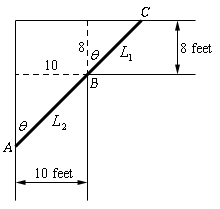The largest pipe that can go around the turn will do so in the position shown above. One end will be touching the outer wall of the hall way at $$A$$ and $$C$$ and the pipe will touch the inner corner at $$B$$. Let’s assume that the length of the pipe in the small hallway is $${L_{\,1}}$$ while $${L_{\,2}}$$ is the length of the pipe in the large hallway. The pipe then has a length of $$L = {L_{\,1}} + {L_{\,2}}$$.

Now, if $$\theta = 0$$ then the pipe is completely in the wider hallway and we can see that as $$\theta \to 0$$ the point $$A$$ will move down the vertical wall and the point $$C$$ will move along the horizontal wall closer and closer to the corner and as this happens $$L$$ lengthens and so $$L \to \infty$$ as $$\theta \to 0$$.

Likewise, if $$\theta = {\frac{\pi}{2}}$$ the pipe is completely in the narrow hallway and as $$\theta \to {\frac{\pi}{2}}$$ we also have $$L \to \infty$$ by a similar line of reasoning above for $$\theta \to 0$$.

So, because $$L \to \infty$$as we near the ends of the interval of possible angles somewhere in the interior of the interval, $$0 < \theta < {\frac{\pi}{2}}$$, is an angle that will minimize $$L$$ and oddly enough that is the length that we’re after. The largest pipe that will fit around the turn will in fact be the minimum value of $$L$$.

The constraint for this problem is not so obvious and there are actually two of them. The constraints for this problem are the widths of the hallways. We’ll use these to get an equation for $$L$$ in terms of $$\theta$$ and then we’ll minimize this new equation.

So, using basic right triangle trig we can see that,

${L_{\,1}} = 8\sec \theta \hspace{0.5in}{L_{\,2}} = 10\csc \theta \hspace{0.5in} \Rightarrow \hspace{0.5in}L = 8\sec \theta + 10\csc \theta$

So, differentiating $$L$$ gives,

$L' = 8\sec \theta \tan \theta - 10\csc \theta \cot \theta$

Setting this equal to zero and solving gives,

\begin{align*}8\sec \theta \tan \theta & = 10\csc \theta \cot \theta \\ \frac{{\sec \theta \tan \theta }}{{\csc \theta \cot \theta }} & = \frac{{10}}{8}\\ \frac{{\sin \theta {{\tan }^2}\theta }}{{\cos \theta }} & = \frac{5}{4}\hspace{0.5in} \Rightarrow \hspace{0.5in}{\tan ^3}\theta = 1.25\end{align*}

Solving for $$\theta$$ gives,

$\,\tan \theta = \sqrt{{1.25}}\hspace{0.5in} \Rightarrow \hspace{0.25in}\theta = {\tan ^{ - 1}}\left( {\sqrt{{1.25}}} \right) = 0.8226$

So, if $$\theta = 0.8226$$ radians then the pipe will have a minimum length and will just fit around the turn. Anything larger will not fit around the turn and so the largest pipe that can be carried around the turn is,

$L = 8\sec \left( {0.8226} \right) + 10\csc \left( {0.8226} \right) = 25.4033{\mbox{ feet}}$
Example 6 Two poles, one 6 meters tall and one 15 meters tall, are 20 meters apart. A length of wire is attached to the top of each pole and it is also staked to the ground somewhere between the two poles. Where should the wire be staked so that the minimum amount of wire is used?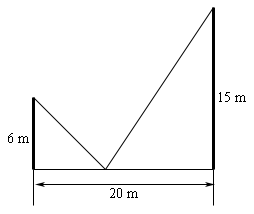Show Solution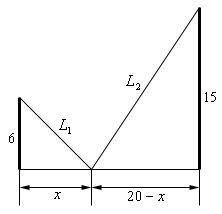The total length of the wire is $$L = {L_{\,1}} + {L_{\,2}}$$ and we need to determine the value of $$x$$ that will minimize this. The constraint in this problem is that the poles must be 20 meters apart and that $$x$$ must be in the range $$0 \le x \le 20$$. The first thing that we’ll need to do here is to get the length of wire in terms of $$x$$, which is fairly simple to do using the Pythagorean Theorem.

${L_{\,1}} = \sqrt {36 + {x^2}} \hspace{0.5in}{L_{\,2}} = \sqrt {225 + {{\left( {20 - x} \right)}^2}} \hspace{0.5in}L = \sqrt {36 + {x^2}} + \sqrt {625 - 40x + {x^2}}$

Not the nicest function we’ve had to work with but there it is. Note however, that it is a continuous function and we’ve got an interval with finite endpoints and so finding the absolute minimum won’t require much more work than just getting the critical points of this function. So, let’s do that. Here’s the derivative.

$L' = \frac{x}{{\sqrt {36 + {x^2}} }} + \frac{{x - 20}}{{\sqrt {625 - 40x + {x^2}} }}$

Setting this equal to zero gives,

\begin{align*}\frac{x}{{\sqrt {36 + {x^2}} }} + \frac{{x - 20}}{{\sqrt {625 - 40x + {x^2}} }} & = 0\\ x\sqrt {625 - 40x + {x^2}} & = - \left( {x - 20} \right)\sqrt {36 + {x^2}} \end{align*}

It’s probably been quite a while since you’ve been asked to solve something like this. To solve this, we’ll need to square both sides to get rid of the roots, but this will cause problems as well soon see. Let’s first just square both sides and solve that equation.

\begin{align*}{x^2}\left( {625 - 40x + {x^2}} \right) & = {\left( {x - 20} \right)^2}\left( {36 + {x^2}} \right)\\ 625{x^2} - 40{x^3} + {x^4} & = 14400 - 1440x + 436{x^2} - 40{x^3} + {x^4}\\ 189{x^2} + 1440x - 14400 & = 0\\ 9\left( {3x + 40} \right)\left( {7x - 40} \right) & = 0\hspace{0.5in} \Rightarrow \hspace{0.5in}x = - \frac{40}{3} ,\,\,\,\,\,x = \frac{40}{7}\end{align*}

Note that if you can’t do that factoring don’t worry, you can always just use the quadratic formula and you’ll get the same answers.

Okay two issues that we need to discuss briefly here. The first solution above (note that we didn’t call it a critical point…) doesn’t make any sense because it is negative and outside of the range of possible solutions and so we can ignore it.

Secondly, and maybe more importantly, if you were to plug $$x = - \frac{40}{3}$$ into the derivative you would not get zero and so is not even a critical point. How is this possible? It is a solution after all. We’ll recall that we squared both sides of the equation above and it was mentioned at the time that this would cause problems. We’ll we’ve hit those problems. In squaring both sides we’ve inadvertently introduced a new solution to the equation. When you do something like this you should ALWAYS go back and verify that the solutions that you get are in fact solutions to the original equation. In this case we were lucky and the “bad” solution also happened to be outside the interval of solutions we were interested in but that won’t always be the case.

So, if we go back and do a quick verification we can in fact see that the only critical point is $$x = \frac{40}{7} = 5.7143$$ and this is nicely in our range of acceptable solutions, $$0 \le x \le 20$$.

Now all that we need to do is plug this critical point and the endpoints of the wire into the length formula and identify the one that gives the minimum value.

$L\left( 0 \right) = 31\hspace{0.5in}L\left( \frac{40}{7} \right) = 29\hspace{0.5in}L\left( {20} \right) = 35.8806$

So, we will get the minimum length of wire if we stake it to the ground $$\frac{40}{7}$$ feet from the smaller pole.

Let’s do a modification of the above problem that asks a completely different question.

Example 7 Two poles, one 6 meters tall and one 15 meters tall, are 20 meters apart. A length of wire is attached to the top of each pole and it is also staked to the ground somewhere between the two poles. Where should the wire be staked so that the angle formed by the two pieces of wire at the stake is a maximum?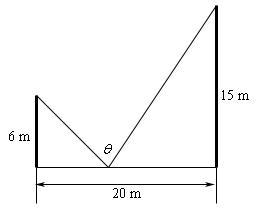Show Solution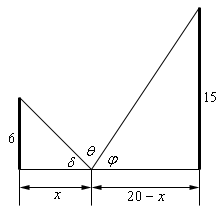The equation that we’re going to need to work with here is not obvious. We can see from the sketch above that,

$\delta + \theta + \varphi = 180 = \pi$

Note that we need to make sure that the equation is equal to $$\pi$$ because of how we’re going to work this problem. Now, basic right triangle trig tells us the following,

$\begin{array}{lll}\tan \delta = \displaystyle \frac{6}{x}& \hspace{0.5in} \Rightarrow \hspace{0.5in} & \delta = {\tan ^{ - 1}}\left( \frac{6}{x}} \right)\\ \tan \varphi = \displaystyle \frac{{15}}{{20 - x}} & \hspace{0.5in} \Rightarrow \hspace{0.5in} & \varphi = {\tan ^{ - 1}}\left( \frac{{15}}{{20 - x}}} \right)\end{array$

Plugging these into the equation above and solving for $$\theta$$ gives,

$\theta = \pi - {\tan ^{ - 1}}\left( {\frac{6}{x}} \right) - {\tan ^{ - 1}}\left( {\frac{{15}}{{20 - x}}} \right)$

Note that this is the reason for the $$\pi$$ in our equation. The inverse tangents give angles that are in radians and so can’t use the 180 that we’re used to in this kind of equation.

Next, we’ll need the derivative so hopefully you’ll recall how to differentiate inverse tangents.

\begin{align*}\theta ' & = - \frac{1}{{1 + {{\left( {{\frac{6}{x}}} \right)}^2}}}\left( { - \frac{6}{{{x^2}}}} \right) - \frac{1}{{1 + {{\left( {{\textstyle{{15} \over {20 - x}}}} \right)}^2}}}\left( {\frac{{15}}{{{{\left( {20 - x} \right)}^2}}}} \right)\\ & = \frac{6}{{{x^2} + 36}} - \frac{{15}}{{{{\left( {20 - x} \right)}^2} + 225}}\\ & = \frac{6}{{{x^2} + 36}} - \frac{{15}}{{{x^2} - 40x + 625}} = \frac{{ - 3\left( {3{x^2} + 80x - 1070} \right)}}{{\left( {{x^2} + 36} \right)\left( {{x^2} - 40x + 625} \right)}}\end{align*}

Setting this equal to zero and solving give the following two critical points.

$x = \frac{- 40 \pm \sqrt {4810}}{3} = - 36.4514,\,\,\,\,\,9.7847$

The first critical point is not in the interval of possible solutions and so we can exclude it.

Clearly $$x$$ must be in the interval $$\left[ {0,20} \right]$$ and so using test points it’s not difficult to show that if $$0 \le x < 9.7847$$ we have $$\theta ' > 0$$ (and so $$\theta$$ is increasing) and if $$9.7847 < x \le 20$$ that $$\theta ' < 0$$ (and so $$\theta$$ is decreasing). So by the first derivative test when $$x = 9.7847$$ we will get the maximum value of $$\theta$$.

Example 8 A trough for holding water is formed by taking a piece of sheet metal 60 cm wide and folding the 20 cm on either end up as shown below. Determine the angle $$\theta$$ that will maximize the amount of water that the trough can hold.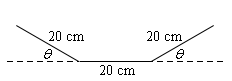Show Solution

Now, in this case we are being asked to maximize the volume that a trough can hold, but if you think about it the volume of a trough in this shape is nothing more than the cross‑sectional area times the length of the trough. So, for a given length in order to maximize the volume all you really need to do is maximize the cross‑sectional area.

To get a formula for the cross-sectional area let’s redo the sketch above a little.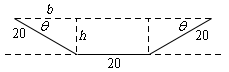We can think of the cross-sectional area as a rectangle in the middle with width 20 and height $$h$$ and two identical triangles on either end with height $$h$$, base $$b$$ and hypotenuse 20. Also note that basic geometry tells us that the angle between the hypotenuse and the base must also be the same angle $$\theta$$ that we had in our original sketch.

Also, basic right triangle trig tells us that the base and height can be written as,

$b = 20\cos \theta \hspace{0.75in}h = 20\sin \theta$

The cross-sectional area for the whole trough, in terms of $$\theta$$, is then,

$A = 20h + 2\left( {{\frac{1}{2}}bh} \right) = 400\sin \theta + \left( {20\cos \theta } \right)\left( {20\sin \theta } \right) = 400\left( {\sin \theta + \sin \theta \cos \theta } \right)$

The derivative of the area is,

\begin{align*}A'\left( \theta \right) & = 400\left( {\cos \theta + {{\cos }^2}\theta - {{\sin }^2}\theta } \right)\\ & = 400\left( {\cos \theta + {{\cos }^2}\theta - \left( {1 - {{\cos }^2}\theta } \right)} \right)\\ & = 400\left( {2{{\cos }^2}\theta + \cos \theta - 1} \right)\\ & = 400\left( {2\cos \theta - 1} \right)\left( {\cos \theta + 1} \right)\end{align*}

So, we have either,

\begin{align*}2\cos \theta - 1 & = 0\hspace{0.5in} \Rightarrow \hspace{0.5in} \cos \theta = {\frac{1}{2}}\,\,\hspace{0.5in} \Rightarrow \hspace{0.5in}\theta = {\frac{\pi}{3}}\\ \cos \theta + 1 & = 0\hspace{0.5in} \Rightarrow \hspace{0.5in}\cos \theta = - 1\hspace{0.5in} \Rightarrow \hspace{0.5in}\theta = \pi \end{align*}

However, we can see that $$\theta$$ must be in the interval $$0 \le \theta \le {\frac{\pi}{2}}$$ or we won’t get a trough in the proper shape. Therefore, the second critical point makes no sense and also note that we don’t need to add on the standard “$$+ \,2\pi n$$” for the same reason.

Finally, since the equation for the area is continuous all we need to do is plug in the critical point and the end points to find the one that gives the maximum area.

$A\left( 0 \right) = 0\hspace{0.5in}A\left( {{\frac{\pi}{3}}} \right) = 519.6152\hspace{0.5in}A\left( {{\frac{\pi}{2}}} \right) = 400$

So, we will get a maximum cross-sectional area, and hence a maximum volume, when $$\theta = {\frac{\pi}{3}}$$.